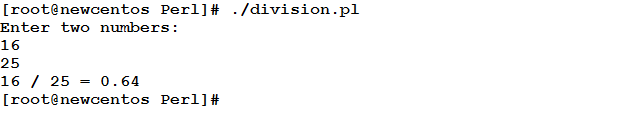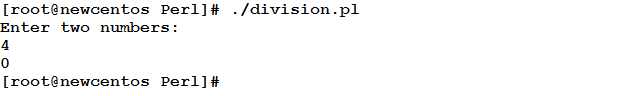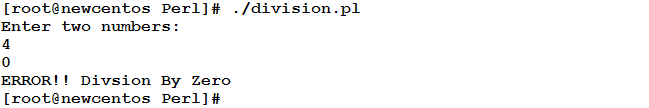# Learn Decision Making in Perl Programming

0
3793Life doesn’t go the same way all the time; at times there will be deviations. Such deviations are forced by the different conditions we face. These conditions control our decisions, and hence our actions. The operation of responding to variable conditions and acting accordingly is called Decision Making. This is going to be the topic of today’s article. Enjoy.

The if Statement
The most popular decision making tool: the if statement. You will never find a programming language that does not have the if statement. The if statement simply is followed by a condition. If the condition evaluates to true, the if code block is executed. If not, nothing executed (this is for now).

Syntax

```if (CONDITION)
{
statement1;
statement2;
…
statementn;
}
```

Where: CONDITION is any Boolean expression that could evaluate to true or false.

Note that the if statement body is delimited by curly braces { }

Example
In this example, we are going to prompt the user to enter two numbers to divide. The script should perform the division operation only if the divisor is not zero.

```#!/usr/bin/perl
#This script accepts two input numbers from the user
#and divides them. the script should execute the division
#only if the divisor is not zero.
#Author: Eduonix
#Date: Oct 2015
print("Enter two numbers:\n");
\$a =  <stdin>; chomp \$a;
\$b =  <stdin>; chomp \$b;
if (\$b != 0)
{
print ("\$a / \$b = ",\$a/\$b,"\n");
}```

Let’s see how this script will behave:Now, what if we enter 0 as the divisor?!Nothing occurs!!

A Short Alternative for Single-Line if Body
In cases like above, where the code block depending on the if statement consists of only one statement, Perl has the following shorter alternative:

`statement 	if (CONDITION);`

So, the if statement in the previous example could be re-written as follows while achieving the same results:

`print ("\$a / \$b = ",\$a/\$b,"\n") 	if (\$b != 0);`

This has eliminated the need for curly braces.

The if…else Statement
In the above example, the division is executed only if the condition is met (\$b is not equal to 0). What if the user has entered wrong input?! Nothing executed. OK, although it seems that we have prevented the illegal division by zero operation, the user doesn’t get notified that he entered illegal input. That is not wrong, but not friendly as well. There should be an alternate route to be followed when the specified condition is not met. The else part solves this problem.

Syntax

```if (CONDITION)
{
statement1;
statement2;
…
statementn;
}
else
{
statement1;
statement2;
…
statementn;
}```

Now, the if statement could look like this:

```if (\$b != 0)
{
print ("\$a / \$b = ",\$a/\$b,"\n");
}
else
{
print ("ERROR!!! Division By Zero\n");
}
```

When the modified script is executed, it should notify the user if he enters an invalid input:if…elsif…else Statement
Now, what if the problem is more complex than just one condition and its alternate route?! i.e. what if there are other conditions to be checked and actions to be decided upon?! In this case, the if…else statement will be insufficient. For this reason, the if…elsif…else structure comes into light.

Syntax

```if (CONDITION)
{
statement1;
statement2;
…
statementn;
}
elsif (CONDITION)
{
statement1;
statement2;
…
statementn;
}
else
{
statement1;
statement2;
…
statementn;
}
```

The unless Statement
Linguistically, the word “unless” is the opposite of “if”. It means if not. Perl keeps for unless its meaning as opposite of if. So, if we want to re-write the division script using unless, one possible form could be:

`print ("\$a / \$b = ",\$a/\$b,"\n") 	unless (\$b == 0);`

The above statement means: execute the division if not \$b is equal to 0.

Note
An important difference between if and unless is that the if body is executed only if the condition evaluates to true. On the other hand, the unless body is executed only if the condition evaluates to false.
That is an important point that should bear in mind.

unless also can have else and elsif parts.

Example
Back again to the sphere volume example. In this modified version, we are going to use the unless statement to validate the user’s input.

Consider the following code:

```#!/usr/bin/perl
#This script prompts the user to enter the radius of sphere.
#and calculate its volume.
#Author: Eduonix
#Date: Nov 2015
print ("Enter the Radius of a sphere: ");
\$r = <stdin>;
chomp \$r;
unless (\$r<=0)
{
\$volume = (4/3) * (3.14) * \$r ** 3;
print("For a sphere with radius \$r : Volume = \$volume\n");
}
elsif (\$r==0)
{    print("Invalid Input: Radius couldn't be Zero\n"); }
elsif (\$r<0)
{    print("Invalid Input: Radius couldn't be a Negative number\n");
}
```

Let’s see how this script will behave:The switch…case structure
Whenever there is a need to test a variable against list of possible values, the switch…case structure could be a good solution. It presents a shorter method than using if…elsif…else with repeated elsif parts for each possible value.

Syntax

```switch(\$var)
{
case VALUE1
{
#execute something
}
case VALUE2
{
#execute something
}
. . .
case VALUEn
{
#execute something
}
else
{
#execute something
}
}```

Note
For the switch…case to work, we need to import the Switch module.

This could be very useful in a menu scripts. In such scripts, a menu of available options is displayed to the user to choose from. Based on the user’s input, the script will display something or make some change. Imagine a menu with 20 options: this will need an if
statement followed by 19 elsif conditions, and all test the possible options against the same variable. This is going to be a quite boring task!!!
Now, consider the switch…case alternative:

```switch (\$choice)
{
case 1
{	#execute something
}
case 2
{	#execute something
}
…
case 20
{	#execute something
}
}```

I think this is much better than repeating the condition (\$choice==x) for x ranging between 1 and 20.

The Conditional Operator
The conditional operator could be a short alternative to an if…else structure with single-statement body for each part.
For example, to check whether a number is odd or even and print the result to the user, we could use the following short form:

`(\$i%2!=0)? print("odd\n"): print("Even\n");`

This is equivalent to saying:

```if (\$i%2!=0)
{
print("odd\n");
}
else
{
print("Even\n");
}```

If you are about to write a similar script, which of the two methods would you choose

Summary
• Decision Making in Perl could be done using either of the following methods:
o The if…elsif…else structure.
o unless…elsif…else structure.
o switch…case structure.
o The Conditional Operator.

In the next article, we are going to talk about Loops. A very interesting topic really, one you should not miss. See you there!!!